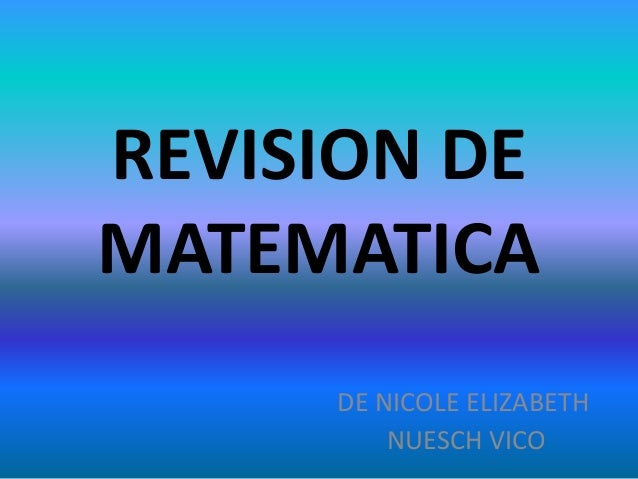Successfully reported this slideshow.Upcoming SlideShare
×

# Revision de matematica nicole nuesch

• Full Name
Comment goes here.

Are you sure you want to Yes No• Be the first to like this

### Revision de matematica nicole nuesch

1. 1. REVISION DE MATEMATICA DE NICOLE ELIZABETH NUESCH VICO
2. 2. PROPIEDADES DE LA POTENCIA • Potencias de exponente 0 Ej. a0 = 1 50 = 1 • Potencias de base 0 Ej. 0n = 0 05 = 0 n>0 • Potencias de exponente 1 Ej. a1 = a 51 = 5 • Potencias de base 1 Ej. 1n = 1 115 = 1 • Potencias de exponente entero negativo • Multiplicación de potencias con la misma base Ej. am · a n = am+n 25 · 22 = 25+2 = 27 • División de potencias con la misma base Ej. am : a n = am – n 25 : 22 = 25 - 2 = 23 • Potencia de otra potencia Ej. (am)n=am · n (25)3 = 215 • Multiplicación de potencias con el mismo exponente Ej. an · b n = (a · b) n 23 · 43 = 83 • División de potencias con el mismo exponente Ej. an : b n = (a : b) n 63 : 33 = 23 La potencia no se distribuye ni con la suma, ni con la resta
3. 3. PROPIEDADES DE LA RAIZ • Raíz de raíz • Distributivita respecto de la multiplicación • Distributivita respecto de la división • Simplificación de índices • Eliminación del radical • Amplificación de índices La raíz no se distribuye ni con la suma, ni con la resta
4. 4. RADICALES Extracción de factores de un radical Existen factores, dentro de un radical, q pueden ser extraídos si el exponente de los mismos es mayor o a lo sumo igual que el índice de la raíz. Para ello deben aplicarse las propiedades de la potenciación y la radicación. Radicales semejantes Dos radicales son semejantes cuando tienen el mismo índice y el mismo radicando.
5. 5. RADICALES, ADICION Y SUSTRACCION Solo es posible sumar o restar términos q contienen radicales semejantes. Existen casos en los cuales ciertos radicales son semejantes luego de llevarlos a su mínima expresión.
6. 6. MULTIPLICACION Y DIVISION DE RADICALES 1° CASO: INDICE IGUAL Para multiplicar y dividir radicales debemos buscar que tenga igual indice. 2°CASO : DIFERENTES INDICES
7. 7. PROPIEDADES DE LA MULTIPLICACION Y LA DIVISION MULTIPLICACION DIVISION CONMUTATIVA a.b = b.a NO ASOCIATIVA a(b.c) = (a.b)c NO DISTRIBUTIVA (a+b).c = a.c+b.c a(b+c) = a.b+a.c Solo a derecha (a+b):c = a:c+b:c c:(a+b) no NEUTRO a.1 = 1.a = a Solo a derecha a:1 = a ABSORVENTE a.0 = 0.a = 0 Solo a izquierda 0:a = 0
8. 8. RACIONALIZACION DE DENOMINADORES Racionalizar el denominador de una fracción es transformarlo en un numero racional; por lo tanto, siempre que en el mismo aparezcan radicales irracionales, se debe hallar una fracción equivalente a la dada con denominador racional. PRIMER CASO : en el denominador hay un único radical con índice igual a 2. Para racionalizar este tipo de expresiones, se debe amplificar por la misma raíz que tiene el denominador.
9. 9. RACIONALIZACION DE DENOMINADORES SEGUNDO CASO : en el denominador hay un único radical con índice mayor que 2. Para racionalizar este tipo de expresiones, se debe amplificar por una raíz que tenga el mismo índice que la raíz de el denominador, cuyo radicando tenga los mismos factores, pero con exponente igual a la diferencia entre el índice y el exponente dado TERCER CASO: el denominador es una suma o resta de uno o dos radicales de índice 2. Para racionalizar este tipo de expresiones, se debe aplicar el producto de una suma de dos términos por su diferencia.
10. 10. CUBO DE UN BINOMIO • Binomio de suma al cubo Un binomio al cubo (suma) es igual al cubo del primero, más el triple del cuadrado del primero por el segundo, más el triple del primero por el cuadrado del segundo, más el cubo del segundo. (a + b)3 = a3 + 3 · a2 · b + 3 · a · b2 + b3 (x + 3)3 = x 3 + 3 · x2 · 3 + 3 · x· 32 + 33 = = x3 + 9x2 + 27x + 27 • Binomio de resta al cubo Un binomio al cubo (resta) es igual al cubo del primero, menos el triple del cuadrado del primero por el segundo, más el triple del primero por el cuadrado del segundo, menos el cubo del segundo. (a − b)3 = a3 − 3 · a2 · b + 3 · a · b2 − b3 (2x − 3)3 = (2x)3 − 3 · (2x)2 ·3 + 3 · 2x· 32 − 33 = = 8x 3 − 36 x2 + 54 x − 27